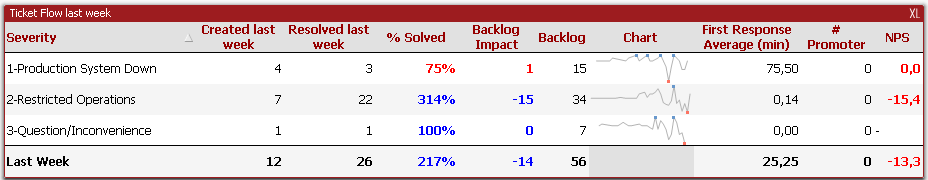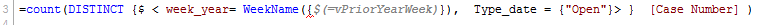# QlikView App Dev

Discussion Board for collaboration related to QlikView App Development.

Announcements
Skip the ticket, Chat with Qlik Support instead for instant assistance.
cancel
Showing results for
Did you mean:Not applicable

## WeekName format with set analysis formula

Hi,

Type_date,

date,

Hour(date) as Hour_date,

date(date) as Date_value,

Day(date) AS  calendar_date,

WeekDay(date) AS  weekday,

Week(date) AS  week,

Month(date) AS  Month,

'Q' & Ceil(Month(date)/3) AS  quarter,

Year(date) AS  year,

// Calendar Date Names

WeekName(date) as  week_year,

MonthName(date) as  month_year,

QuarterName(date) as  quarter_year

FROM

[Dates.qvd]

(qvd);

I created variables like this, with the field called 'date' :

let vMaxYearWeek  = '=WeekName(max(date))';

let vPriorYearWeek  = '=vMaxYearWeek-1';

let vMaxWeek  = '=week(max(date))';

let vPriorWeek  = '=vMaxWeek-1';

Then i used this in a table :Foir 'cteated last week' column :

=count(DISTINCT {\$ < week= {\$(=vPriorWeek)},  Type_date = {"Open"}> }  [Case Number] )

And it works well, but I realized it wasn't the right format date as i could have data from the same week but different year. I have to use year-week.

But if i use this one, I have got '0' value :

=count(DISTINCT {\$ < week_year= {\$(=vPriorYearWeek)},  Type_date = {"Open"}> }  [Case Number] )

I checked results with text object like this :

=vMaxWeek : result is 3

=vPriorWeek : result is 2

=vMaxYearWeek   result is 2017/03

=vPriorYearWeek result is 42750

So i change the last case like this :

=WeekName(vPriorYearWeek) and the result is 2017/02. What I wanted

So if i change table formula with weekname format, it doesn't works.

=count(DISTINCT {\$ < week_year= WeekName({\$(=vPriorYearWeek)}),  Type_date = {"Open"}> }  [Case Number] )

after weekname, bracket is underlined by redWhat have I to do in order to change the format of the variable matching 2017/02 and not 42750 with analysis formula?

PS : i useThanks

Stephane

1 Solution

Accepted SolutionsMVP

week_year = {"\$(=WeekName(\$(vPriorYearWeek)))"}

Next I thin, it would be better to create your vPriorYearWeek Variable like this

let vPriorYearWeek  = '=WeekName(max(date)-7)';

and then use this

week_year = {"\$(=\$(vPriorYearWeek))"}

or

week_year = {"\$(=vPriorYearWeek)"}

4 RepliesMVP
 jeanneteau stephane wrote: after weekname, bracket is underlined by redWhat are you trying? Added Double quotes. What is the use to declare the WeekName here and what was the variable you wriiten

=count(DISTINCT {\$ < week_year= {"\$(=vPriorYearWeek)"},  Type_date = {"Open"}> }  [Case Number] )

What have I to do in order to change the format of the variable matching 2017/02 and not 42750 with analysis formula?

For this, Use WeekYear(FieldName) & '/' & Week(FieldName)

OR

WeekName(\$(VariableName))

Before develop something, think If placed (The Right information | To the right people | At the Right time | In the Right place | With the Right context)MVP

week_year = {"\$(=WeekName(\$(vPriorYearWeek)))"}

Next I thin, it would be better to create your vPriorYearWeek Variable like this

let vPriorYearWeek  = '=WeekName(max(date)-7)';

and then use this

week_year = {"\$(=\$(vPriorYearWeek))"}

or

week_year = {"\$(=vPriorYearWeek)"}Not applicable
Author

You're right, but i had also to change the creation of my variable like Sunny explained below :

let vPriorYearWeek  = '=WeekName(max(date)-7)';

With you're advise and Sunny too, i succed to fix my issue

thank youNot applicable
Author

Thank you

It's resolved nowCommunity Browser